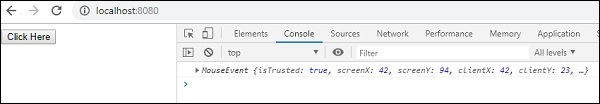# RxJS - Filtering Operator skip

This operator will give back an observable that will skip the first occurrence of count items taken as input.

## Syntax

```skip(count: number): Observable
```

## Parameters

count − The argument count is the number of times that the items will be skipped from the source observable.

## Return value

It will return an observable that skips values based on the count given.

## Example

```import { fromEvent, interval } from 'rxjs';

import { skip} from 'rxjs/operators';

let btn = document.getElementById("btnclick");
let btn_clicks = fromEvent(btn, 'click');
let case1 = btn_clicks.pipe(skip(2));
case1.subscribe(x => console.log(x));
```

We have given count as 2 to skip() operator so the first two clicks are ignored and the third click event is emitted.

## Output